# Problem 51471. Find integer solutions to an equation with a sum of binomial coefficients

Theorem 1 of chapter 27 of the book Diophantine Equations by Louis J. Mordell shows that the equationhas a finite number of real, integer solutions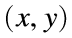. One of them has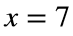because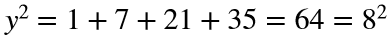.
Write a function that takes a value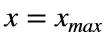­ and returns a two-column matrix withpairs for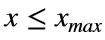. Sort the pairs in order of increasing x.

### Solution Stats

30.43% Correct | 69.57% Incorrect
Last Solution submitted on Aug 11, 2021

### Community Treasure Hunt

Find the treasures in MATLAB Central and discover how the community can help you!

Start Hunting!